Home »

# HTML Output

## HTML Output Tag

The `<output>` tag represents the result of a calculation.

<!DOCTYPE html>
<html lang="en">
<meta charset="UTF-8">
<title> HTML Output Tag </title>
<body>
<form oninput="x.value=parseInt(a.value)+parseInt(b.value)">0
<input type="range" id="a" value="50">100
+<input type="number" id="b" value="50">
=<output name="x" for="a b">
</output>
</form>
</body>
</html>

HTML Output Tag
0 100 + =

### HTML Output Attribute

Attribute Description
for Defines the relationship between the result of the calculation, and the elements used in the calculation.
form Specifies one or more forms the output element belongs to.
name Define a name for the output element.

### Browser Support

Element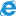Edge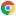Chrome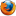Firefox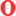Opera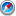Safari
`<output>`
Yes
Yes
Yes
Yes
Yes

PHP Introduction

How to Run a PHP program in Xampp?

How can I create a phpinfo.php page?

How to Define a Variable in PHP

PHP Echo and Print Statements

Working with PHP Operators

Understanding the PHP Data Types

How to Write Comments in PHP

How to Define a Constant in PHP

PHP if...else...elseif Statements